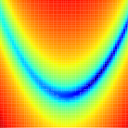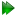Subsections

minimise.executeSynopsis

Perform an optimisation.

Defaults

minimise.execute(min_algor=`newton', line_search=None, hessian_mod=None, hessian_type=None, func_tol=1e-25, grad_tol=None, max_iter=10000000, constraints=True, scaling=True, verbosity=1)

Keyword arguments

min_algor: The optimisation algorithm to use.

line_search: The line search algorithm which will only be used in combination with the line search and conjugate gradient methods. This will default to the More and Thuente line search.

hessian_mod: The Hessian modification. This will only be used in the algorithms which use the Hessian, and defaults to Gill, Murray, and Wright modified Cholesky algorithm.

hessian_type: The Hessian type. This will only be used in a few trust region algorithms, and defaults to BFGS.

func_tol: The function tolerance. This is used to terminate minimisation once the function value between iterations is less than the tolerance. The default value is 1e-25.

grad_tol: The gradient tolerance. Minimisation is terminated if the current gradient value is less than the tolerance. The default value is None.

max_iter: The maximum number of iterations. The default value is 1e7.

constraints: A boolean flag specifying whether the parameters should be constrained. The default is to turn constraints on (constraints=True).

scaling: The diagonal scaling boolean flag. The default that scaling is on (scaling=True).

verbosity: The amount of information to print to screen. Zero corresponds to minimal output while higher values increase the amount of output. The default value is 1.

Description

This will perform an optimisation starting from the current parameter values. This is only suitable for data pipe types which have target functions and hence support optimisation.

Diagonal scaling

Diagonal scaling is the transformation of parameter values such that each value has a similar order of magnitude. Certain minimisation techniques, for example the trust region methods, perform extremely poorly with badly scaled problems. In addition, methods which are insensitive to scaling such as Newton minimisation may still benefit due to the minimisation of round off errors.

In Model-free analysis for example, if S2 = 0.5, τe = 200 ps, and Rex = 15 1/s at 600 MHz, the unscaled parameter vector would be [0.5, 2.0e-10, 1.055e-18]. Rex is divided by (2 * π * 600,000,000)**2 to make it field strength independent. The scaling vector for this model may be something like [1.0, 1e-9, 1/(2 * π * 6e8)**2]. By dividing the unscaled parameter vector by the scaling vector the scaled parameter vector is [0.5, 0.2, 15.0]. To revert to the original unscaled parameter vector, the scaled parameter vector and scaling vector are multiplied.

Minimisation algorithms

A minimisation function is selected if the minimisation algorithm matches a certain pattern. Because the python regular expression `match' statement is used, various strings can be supplied to select the same minimisation algorithm. Below is a list of the minimisation algorithms available together with the corresponding patterns.

This is a short description of python regular expression, for more information, see the regular expression syntax section of the Python Library Reference. Some of the regular expression syntax used in this function is:

`[]' -
A sequence or set of characters to match to a single character. For example, `[Nn]ewton' will match both `Newton' and `newton'.
`^' -
Match the start of the string.
`\$' -
Match the end of the string. For example, `^[Ll][Mm]\$' will match `lm' and `LM' but will not match if characters are placed either before or after these strings.

To select a minimisation algorithm, use a string which matches one of the following patterns given in the tables.

Unconstrained line search methods:

Please see Table 17.14 on page.

 Minimisation algorithm Patterns Back-and-forth coordinate descent '^[Cc][Dd]\$' or '^[Cc]oordinate[ _-][Dd]escent\$' Steepest descent '^[Ss][Dd]\$' or '^[Ss]teepest[ _-][Dd]escent\$' Quasi-Newton BFGS '^[Bb][Ff][Gg][Ss]\$' Newton '^[Nn]ewton\$' Newton-CG '^[Nn]ewton[ _-][Cc][Gg]\$' or '^[Nn][Cc][Gg]\$'

Unconstrained trust-region methods:

Please see Table 17.15 on page.

 Minimisation algorithm Patterns Cauchy point '^[Cc]auchy' Dogleg '^[Dd]ogleg' CG-Steihaug '^[Cc][Gg][-_ ][Ss]teihaug' or '^[Ss]teihaug' Exact trust region '^[Ee]xact'

Please see Table 17.16 on page.

 Minimisation algorithm Patterns Fletcher-Reeves '^[Ff][Rr]\$' or '^[Ff]letcher[-_ ][Rr]eeves\$' Polak-Ribiere '^[Pp][Rr]\$' or '^[Pp]olak[-_ ][Rr]ibiere\$' Polak-Ribière + '^[Pp][Rr] \+\$' or '^[Pp]olak[-_ ][Rr]ibiere \+\$' Hestenes-Stiefel '^[Hh][Ss]\$' or '^[Hh]estenes[-_ ][Ss]tiefel\$'

Miscellaneous unconstrained methods:

Please see Table 17.17 on page.

 Minimisation algorithm Patterns Simplex '^[Ss]implex\$' Levenberg-Marquardt '^[Ll][Mm]\$' or '^[Ll]evenburg-[Mm]arquardt\$'

Global minimisation methods:

Please see Table 17.18 on page.

 Minimisation algorithm Patterns Simulated Annealing '^[Ss][Aa]\$' or '^[Ss]imulated [Aa]nnealing\$'

Minimisation options

The minimisation options can be given in any order.

Line search algorithms. These are used in the line search methods and the conjugate gradient methods. The default is the Backtracking line search. The algorithms are:

Please see Table 17.19 on page.

 Line search algorithm Patterns Backtracking line search '^[Bb]ack' Nocedal and Wright interpolation based line search '^[Nn][Ww][Ii]' or '^[Nn]ocedal[ _][Ww]right[ _][Ii]nt' Nocedal and Wright line search for the Wolfe conditions '^[Nn][Ww][Ww]' or '^[Nn]ocedal[ _][Ww]right[ _][Ww]olfe' More and Thuente line search '^[Mm][Tt]' or '^[Mm]ore[ _][Tt]huente\$' No line search '^[Nn]o [Ll]ine [Ss]earch\$'

Hessian modifications. These are used in the Newton, Dogleg, and Exact trust region algorithms:

Please see Table 17.20 on page.

 Hessian modification Patterns Unmodified Hessian '^[Nn]o [Hh]essian [Mm]od' Eigenvalue modification '^[Ee]igen' Cholesky with added multiple of the identity '^[Cc]hol' The Gill, Murray, and Wright modified Cholesky algorithm '^[Gg][Mm][Ww]\$' The Schnabel and Eskow 1999 algorithm '^[Ss][Ee]99'

Hessian type, these are used in a few of the trust region methods including the Dogleg and Exact trust region algorithms. In these cases, when the Hessian type is set to Newton, a Hessian modification can also be supplied as above. The default Hessian type is Newton, and the default Hessian modification when Newton is selected is the GMW algorithm:

Please see Table 17.21 on page.

 Hessian type Patterns Quasi-Newton BFGS '^[Bb][Ff][Gg][Ss]\$' Newton '^[Nn]ewton\$'

For Newton minimisation, the default line search algorithm is the More and Thuente line search, while the default Hessian modification is the GMW algorithm.

Prompt examples

To apply Newton minimisation together with the GMW81 Hessian modification algorithm, the More and Thuente line search algorithm, a function tolerance of 1e-25, no gradient tolerance, a maximum of 10,000,000 iterations, constraints turned on to limit parameter values, and have normal printout, type any combination of:

[numbers=none]
relax> minimise.execute('newton')

[numbers=none]
relax> minimise.execute('Newton')

[numbers=none]
relax> minimise.execute('newton', 'gmw')

[numbers=none]
relax> minimise.execute('newton', 'mt')

[numbers=none]
relax> minimise.execute('newton', 'gmw', 'mt')

[numbers=none]
relax> minimise.execute('newton', 'mt', 'gmw')

[numbers=none]
relax> minimise.execute('newton', func_tol=1e-25)

[numbers=none]

[numbers=none]
relax> minimise.execute('newton', max_iter=1e7)

[numbers=none]
relax> minimise.execute('newton', constraints=True, max_iter=1e7)

[numbers=none]
relax> minimise.execute('newton', verbosity=1)

To use constrained Simplex minimisation with a maximum of 5000 iterations, type:

[numbers=none]
relax> minimise.execute('simplex', constraints=True, max_iter=5000)

The relax user manual (PDF), created 2020-08-26.